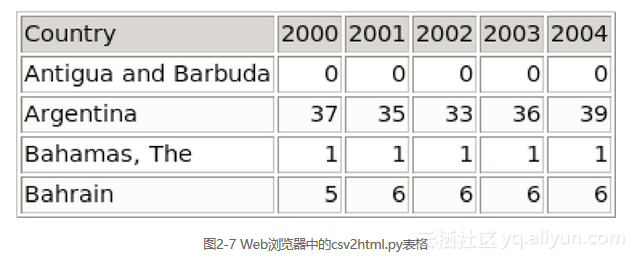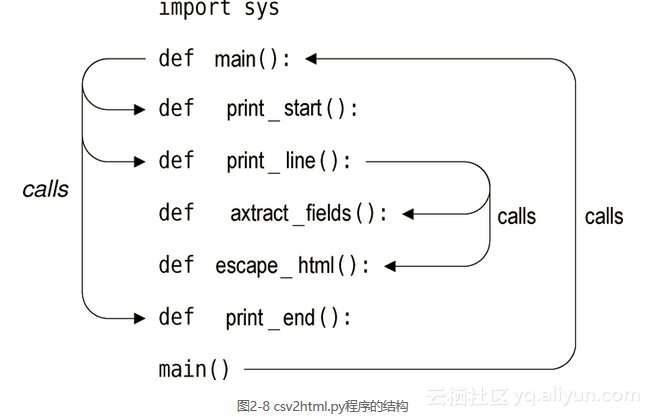《Python 3程序开发指南（第2版•修订版）》——2.5 实例

+关注继续查看

2.5 实例

ax^2 + bx + c = 0
enter a: 2.5
enter b: 0
enter c: -7.25
2.5x^2 + 0.0x + -7.25 = 0 → x = 1.70293863659 or x = -1.70293863659

1.5x^2 + -3.0x + 6.0 = 0 → x = (1+1.7320508j)或x = (1-1.7320508j)

import cmath
import math
import sys

def get_float(msg, allow_zero):
x = None
while x is None:
try:
x = float(input(msg))
if not allow_zero and abs(x) < sys.float_info.epsilon:
print("zero is not allowed")
x = None
except ValueError as err:
print(err)
return x

print("ax\N{SUPERSCRIPT TWO} + bx + c = 0")
a = get_float("enter a: ", False)
b = get_float("enter b: ", True)
c = get_float("enter c: ", True)

x1 = None
x2 = None
discriminant = (b ** 2) - (4 * a * c)
if discriminant == 0:
x1 = -(b / (2 * a))
else:
if discriminant > 0:
root = math.sqrt(discriminant)
else: # discriminant < 0
root = cmath.sqrt(discriminant)
x1 = (-b + root) / (2 * a)
x2 = (-b - root) / (2 * a)

equation = ("{0}x\N{SUPERSCRIPT TWO} + {1}x + {2} = 0"
" \N{RIGHTWARDS ARROW} x = {3}").format(a, b, c, x1)
if x2 is not None:
equation += " or x = {0}".format(x2)
print(equation)

equation = ("{a}x\N{SUPERSCRIPT TWO} + {b}x + {c} = 0"
" \N{RIGHTWARDS ARROW} x = {x1}").format(**locals())

equation = ("{}x\N{SUPERSCRIPT TWO} + {}x + {} = 0"
" \N{RIGHTWARDS ARROW} x = {}").format(a, b, c, x1)

2.5.2 csv2html.py

CSV格式每行一个记录，每个记录使用逗号分隔为多个字段。每个字段可以是字符串，也可以是数字。字符串必须使用单引号或双引号包含起来，数字不应该使用引号包含，除非其中包含逗号。在字符串内部使用逗号是允许的，但不能充当字段分隔符。我们假定第一条记录包含字段labels。我们将要产生的输出是HTML表格，其中的文本采用左对齐方式（在HTML中是默认的），数字则采用右对齐方式，每个记录一列，每个字段一个单元。

"COUNTRY","2000","2001",2002,2003,2004
"ANTIGUA AND BARBUDA",0,0,0,0,0
"ARGENTINA",37,35,33,36,39
"BAHAMAS, THE",1,1,1,1,1
"BAHRAIN",5,6,6,6,6

<table border='1'><tr bgcolor='lightgreen'>
<td>Country</td><td align='right'>2000</td><td align='right'>2001</td>
<td align='right'>2002</td><td align='right'>2003</td>
<td align='right'>2004</td></tr>
...
<tr bgcolor='lightyellow'><td>Argentina</td>
<td align='right'>37</td><td align='right'>35</td>
<td align='right'>33</td><td align='right'>36</td>
<td align='right'>39</td></tr>
...
</table>main()Python读入文件时，从顶部开始执行，这一实例也是如此，首先执行的是导入语句，之后创建了main()函数，再之后创建了其他函数，其顺序与文件中出现的顺序一致。在文件尾部调用main()函数时，main()函数要调用的所有函数（以及这些函数要调用的函数）都已经存在。执行过程与我们通常认为的一样，从对main()的调用开始。

def main():
maxwidth = 100
print_start()
count = 0
while True:
try:
line = input()
if count == 0:
color = "lightgreen"
elif count % 2:
color = "white"
else:
color = "lightyellow"
print_line(line, color, maxwidth)
count += 1
except EOFError:
break
print_end()

maxwidth变量用于限制每个cell中的字符数——如果某个字段大于这个值，我们将对其削减，并通过添加省略号来表明这一点。我们下面就开始查看print_start()、print_line()以及print_end()等函数，while循环对每行输入进行迭代处理——输入可以来自用户的键盘输入，但是我们更希望来自重定向文件。我们设置了想要使用的颜色，并调用print_line()将该行以HTML表格列的形式输出。

def print_start():
print("<table border='1'>")
def print_end():
print("</table>")

def print_line(line, color, maxwidth):
print("<tr bgcolor='{0}'>".format(color))
fields = extract_fields(line)
for field in fields:
if not field:
print("<td></td>")
else:
number = field.replace(",", "")
try:
x = float(number)
print("<td align='right'>{0:d}</td>".format(round(x)))
except ValueError:
field = field.title()
field = field.replace(" And ", " and ")
if len(field) <= maxwidth:
field = escape_html(field)
else:
field = "{0} ...".format(
escape_html(field[:maxwidth]))
print("<td>{0}</td>".format(field))
print("</tr>")

def extract_fields(line):
fields = []
field = ""
quote = None
for c in line:
if c in "\"'":
if quote is None: # start of quoted string
quote = c
elif quote == c: # end of quoted string
quote = None
else:
field += c # other quote inside quoted string
continue
if quote is None and c == ",": # end of a field
fields.append(field)
field = ""
else:
field += c            # accumulating a field
if field:
fields.append(field)  # adding the last field
return fields

def escape_html(text):
text = text.replace("&", "&amp;")
text = text.replace("<", "&lt;")
text = text.replace(">", "&gt;")
return text《Abaqus GUI程序开发指南（Python语言）》——2.2　Python与Abaqus
4323 0python用zipfile模块打包文件或是目录、解压zip文件实例
863 0python 抓取分析 SGMLParser 实例
1029 0ORA-07445导致实例崩溃的解决【The solution of instance crush by ORA-07445】
779 0《Abaqus GUI程序开发指南（Python语言）》——1.2　Abaqus图形界面程序开发的意义
2270 0《Python语言程序设计》——2.13　软件开发流程
1318 0if/while/for 、解决数学难题 、 Python实例
649 0《树莓派开发实战（第2版）》——2.2　查看自己的IP地址
1506 0《Abaqus GUI程序开发指南（Python语言）》——第一章 概述1.1　简单插件实例——创建带孔板有限元模型
2584 0《Web异步与实时交互——iframe AJAX WebSocket开发实战》—— 2.3　示例程序
1563 0

12049

0

《2021云上架构与运维峰会演讲合集》

《零基础CSS入门教程》

《零基础HTML入门教程》